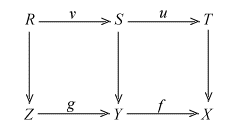1.1. Strong Monos  In this section we recall some basic properties of strong monos in a category with pullbacks. Please note that the notion of a strong mono is also defined for a general category (cf. Adamek, Herrlich and Strecker 1990] or [Borceux 1994 Vol I]). However, for our purpose the following simplified approach is sufficient.  Definition 1.1.1. (a) A strong mono is a map such that any of its pullbacks is not non-isomorphic epic.  (b) A regular mono is a map which can be written as the equalizer of some pair of maps.  Proposition 1.1.2. (a) Any strong mono is a mono.  (b) An epic strong mono is an isomorphism.  (c) Any pullback of a strong mono is a strong mono.  (d) Any composite of strong monos is a strong mono.  (e) Any intersection of strong monos is a strong mono.  Proof. (a) If f: Y ® X is not a mono then its pullback along itself is a non-isomorphic epi (a retraction). Thus f is not a strong mono.  (b) and (c) by definition.  (d) Suppose f: Y ® X and g: Z ® Y are two strong monos. Suppose an epi w: R ® T is the pullback of f°g along a map t: T ® X. We have to prove that w is an isomorphism. Let u: S ® T be the pullback of f along t. Then w factors through u in a map v: R ® S, and u is an epi because w is so. Then u is an isomorphism as f is a strong mono. From w = u°v we conclude that v is an epi. But g is a strong mono and v is a pullback of g. So v is also an isomorphism. This shows that w = u°v is an isomorphism.(e) Suppose f is an intersection of strong monos {fi}. The pullback g of f along a map is the intersection of the pullbacks {gi} of {fi} along the same map. If g is epic then each gi is also epic as g factors through gi; thus each gi is an isomorphism as fi is a strong mono. Hence g as an intersection of isomorphisms is an isomorphism. This shows that f is a strong mono. n    Proposition 1.1.3.  (a) Any pullback of regular mono is a regular mono.  (b) A map is not epic iff it factors through a non-isomorphic regular mono.  (c) Any regular mono is a strong mono.  (d) Any non-isomorphic strong mono factors through a non-isomorphic regular mono.  (e) A map is not epic iff it factors through a non-isomorphic strong mono.  Proof.  (a) If u: U ® X is the equalizer of a pair of maps (s, t): X ® Z and f: Y ® X is any map then the pullback of u along f is the equalizer of (s°f, t°f).  (b) A map f: Y ® X is not epic iff there is a pair of different maps (s, t): X ® Z such that s°f = t°f , in which case f factors through the equalizer of (s, t), which is a non-isomorphic regular mono.  (c) A regular mono is not non-isomorphic epic by (b) and any pullback of a regular mono is a regular mono by (a).  (d) follows from (b) as any non-isomorphic strong mono is not epic.  (e) follows from (b) - (d). n  If a map f: Y ® X in a category is the composite m°e of an epi e: Y ® T followed by a strong (resp. regular) mono m: T ® X  then the pair (e, m) is called an epi-strong-mono (resp. epi-reg-mono) factorization of f; the codomain T of e is called the strong (resp. regular)  image of f. We say a category has epi-strong-mono (resp. epi-regular-mono) factorizations if any map has such a factorization.  Proposition 1.1.4.  (a) Suppose f: Y ® X is a map which is the composite of a map e: Y ® U and a strong mono m: U ® X. Then e is epic iff m is the smallest strong mono to X which f factors through.  {b} Assume any composite of regular monos is regular. Suppose f: Y ® X is a map which is the composite of a map e: Y ® U and a regular mono m: U ® X. Then e is epic iff m is the smallest regular mono to X which f factors through.  {c} An epi-strong-mono (resp. epi-reg-mono) factorization of a map is unique up to isomorphism.  Proof. (a) follows from (1.1.2.d) and (1.1.3.e); (b) can be proved similarly using (1.1.3.b); (c) follows form (a) and (b). n    Proposition 1.1.5. Consider a category A with pullbacks.  (a) A has epi-strong-mono factorizations if any intersection of strong monos exists.  (b) A has epi-regular-mono factorizations if the class of regular monos is closed under composition and intersection.  (c) A has epi-regular-mono factorizations if any composite of regular monos is a regular mono and any map has a cokernel pair.  (d) If A has epi-regular-mono factorizations then any strong mono is regular.    Proof.  (a) Any map f: Y ® X can be factored as a map e: Y ® U followed by a mono m: U ® X, where m is the intersection of all the strong monos in which f can be factored through. Then m is a strong mono by (1.1.2.e) and e is an epi by (1.1.4.a).  (b) Similar to (a).  (c) Any map f: Y ® X can be factored as a map e: Y ® U followed by a regular mono m: U ® X, which is the equalizer of the cokernel pair of f. Then m is the smallest regular mono such that f factors through. Thus e is epic by (1.1.4.b).  (d) follows from (1.1.4.c). n          [Next Section][Content][References][Notations][Home]